##### Trigonometry For DummiesIn some trigonometry problems, you may not know what the measure of an angle is, but you know something about the angle’s function values. For example, suppose you have two angles, α in the second quadrant of a unit circle, and β in the first quadrant.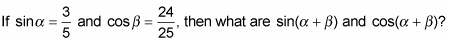1. Find all the necessary function values for the sums.

Both the sine and cosine angle-sum identities use both the sine and cosine of each angle involved. You already know the sine of one angle and the cosine of the other angle, so you have to determine the unknown cosine and sine — you can do so by using the Pythagorean identity:

• First, use the value for sinα to solve for cosβ: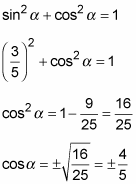You end up with two results. Because the terminal side of angle α is in the second quadrant, the cosine of α, in this case, is negative: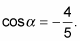• Now use the value for cosβ to solve for sinβ: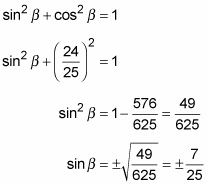The terminal side of angle β is in the first quadrant, where the sine is positive: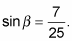2. Insert the function values into the identities for the sine and cosine of the sum of angles.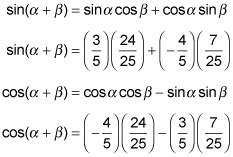3. Simplify the identities and solve for the answers.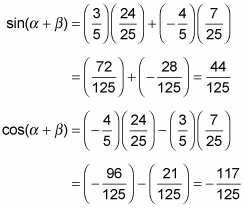By looking at the angle measures, you can predict whether the function value will be positive or negative. In the preceding example, the smaller angles, when added together, create an angle with its terminal side in the second quadrant. The sine of an angle in the second quadrant is positive. So it’s no surprise that the sine comes out to be a positive value and, likewise, that the cosine is a negative value (because cosine is negative in the second quadrant).